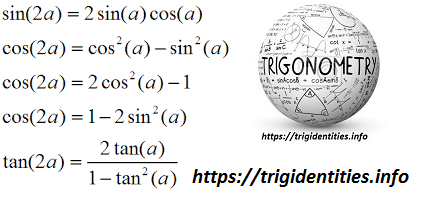# Trig Double Identities

The Trigonometric Double Angle identities or Trig Double identities actually deals with the double angle of the trigonometric functions. For instance,

1. Sin2(α)
2. Cos2(α)
3. Tan2(α)
4. Cosine2(α)
5. Sec2(α)
6. Cot2(α)

Double Angle identities are a special case of trig identities where the double angle is obtained by adding 2 different angles. In this article, we will cover up the different aspects of Trig Double Identities. Before Double, You must have an understanding of Trig Basic Identities.## Trig Double Identities – Trigonometric Double Angle Identities

Here are some of the formulas which are expressing the trigonometric double angled identities in terms of angle x.

 Sin(2x) = 2sinxcosx Cos(2x) = Cosˆ2x – Sinˆ2x = 2cosˆ2x -1 = 1- 2sinˆx 1 Tan(2x) = 2tanx/1-tanˆ2x

If you are looking for Trig Hyperbolic Identities for Trig Double Angle identities then here comes. You can also learn about Hyperbolic Trig Identities.

 Sinh (2x) = 2 sinh x cosh x Cosh (2x) = 2coshˆ2 -1 Tanh (2x) = 2 tanh x/1+tanhˆ2x